# Luggage and air travel

Two friends traveling by plane had a total of 35 kg of luggage. They paid one 72 CZK and second 108 CZK for being overweight. If only one paid for all the bags, it would cost 300 CZK. What weight of baggage did each of them have, how many kilograms of luggage could a single passenger carry for free?

Result

a =  16 kg
b =  19 kg
x =  10 kg

#### Solution:

a+b = 35
a-x = 72p
b-x = 108p
a+b-x = 300p

a+b = 35
a-72p-x = 0
b-108p-x = 0
a+b-300p-x = 0

a = 16
b = 19
p = 112 ≈ 0.083333
x = 10

Calculated by our linear equations calculator.

Leave us a comment of example and its solution (i.e. if it is still somewhat unclear...):

Showing 0 comments:Be the first to comment!#### To solve this example are needed these knowledge from mathematics:

Do you have a system of equations and looking for calculator system of linear equations?

## Next similar examples:

1. Three friendsThe three friends spent 600 KC in a teahouse. Thomas paid twice as much as Paul. Paul a half less than Zdeněk. How many each paid?
2. Freight train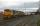The train carries 525 tons of limestone in 29 wagons. Wagons are 15 tonne and 20 tons. How many is 15 ton and how many is 20 ton wagons?
3. Three brothersThe three brothers have a total of 42 years. Jan is five years younger than Peter and Peter is 2 years younger than Michael. How many years has each of them?
4. Collecting day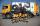At collecting day at school pupils of three classes took total of 1063 kg of paper. Class B collected half more than A Class and class C 55 kg more than B. How many kg of paper collected each class?
5. Two numbersWe have two numbers. Their sum is 140. One-fifth of the first number is equal to half the second number. Determine those unknown numbers.
6. Three daysDuring the three days sold in stationery 1490 workbooks. The first day sold about workbooks more than third day. The second day 190 workbooks sold less than third day. How many workbooks sold during each day?
7. Three granaries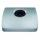Three granaries hold 7,800 metric cent (q) of grain. In the first, 70 tonnes of grain was more than the second and in the third it was 120 tonnes less than in the second. How many grain were in each granary?
8. EquationsSolve following system of equations: 6(x+7)+4(y-5)=12 2(x+y)-3(-2x+4y)=-44
9. Hotel roomsIn the 45 rooms, there were 169 guests, some rooms were three-bedrooms and some five-bedrooms. How many rooms were?
10. Barrel 4Barrel of water weighs 63 kg. After off 75% water, the weight of the barrel with water is 21 kg. How many kg weigh empty barrel and how many kgs water in it?
11. MushroomsEva and Jane collected 114 mushrooms together. Eve found twice as much as Jane. How many mushrooms found each of them?
12. Equations - simpleSolve system of linear equations: x-2y=6 3x+2y=4
13. Tunnels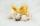Mice had built an underground house consisting of chambers and tunnels: • each tunnel leading from the chamber to the chamber (none is blind) • from each chamber lead just three tunnels into three distinct chambers, • from each chamber mice can get to any
14. TreesAlong the road were planted 250 trees of two types. Cherry for 60 CZK apiece and apple 50 CZK apiece. The entire plantation cost 12,800 CZK. How many was cherries and apples?
15. TheatroTheatrical performance was attended by 480 spectators. Women were in the audience 40 more than men and children 60 less than half of adult spectators. How many men, women and children attended a theater performance?
16. Linear systemSolve this linear system (two linear equations with two unknowns): x+y =36 19x+22y=720
17. Sweets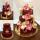3 chocolate and 7 cakes cost 85, - CZK. 2 chocolates and 6 cakes cost 86, - CZK. How much is 5 chocolates and 9 cakes? I wonder how to get the result, but only by logic without the use of a system of equations